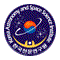# KVN and VERA Array (KaVA)

## Angular Resolution and Largest Detectable Angular Scale

The expected angular resolutions for the K band and the Q band are about 1.2 mas and 0.6 mas, respectively. The synthesized beam size strongly depends on UV coverage, and could be higher than the values mentioned above because the baselines projected on UV plane become shorter than the distance between antennas. The beam size can be calculated approximately by the following formula;(2)

whereandare observed wavelength in centimeter and the maximum baseline length in kilometer, respectively.

The minimum detectable angular scale for interferometers can be also expressed by equation (2), where the baseline lengthis replaced with the shortest one among the array. Because of the relatively short baselines provided by KVN,300 km, KaVA is able to detect an extended structure up to 9 mas and 5 mas for the K and Q bands, respectively.KaVA ( KVN and VERA Array )